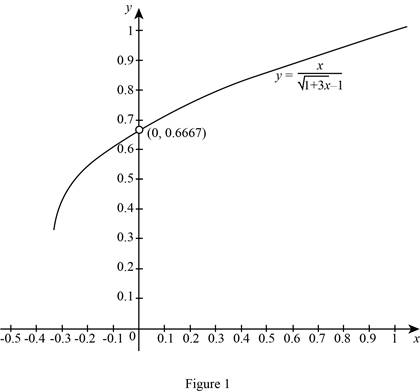# To estimate: The value of the function when x approaches zero by graphing the function f ( x ) .### Single Variable Calculus: Concepts...

4th Edition
James Stewart
Publisher: Cengage Learning
ISBN: 9781337687805### Single Variable Calculus: Concepts...

4th Edition
James Stewart
Publisher: Cengage Learning
ISBN: 9781337687805

#### Solutions

Chapter 2.3, Problem 25E

(a)

To determine

## To estimate: The value of the function when x approaches zero by graphing the function f(x).

Expert Solution

The estimated value of the function when x approaches zero f(x) approaches 0.6667 or 23.

### Explanation of Solution

Given:

The graph of the function f(x)=x1+3x1.

Draw the graph of the function f(x)=x1+3x1 by using the graphing calculator as shown below in Figure 1.From the graph, as x=0, then f(x) is not defined. But x approaches 0, then f(x) goes to 23.

That is, limx0x1+3x123.

Thus, the estimated value of limx0x1+3x1 is 23_.

(b)

To determine

### To guess: The value of the limit by using the table of values of f(x) for x close to 0.

Expert Solution

The value of the limit by using the table of values of f(x) for x close to 0 is 23_.

### Explanation of Solution

Calculation:

Make the table of values of f(x) for x close to 0.

 x 1+3x−1 f(x)=x1+3x−1 −0.001 1+3(−0.001)−1                   ≈−0.0015011267 −0.001−0.0015011267≈0.666 166 3 −0.000 1 1+3(−0.0001)−1                   ≈−0.000150011 −0.0001−0.000150011≈0.666 6167 −0.000 01 1+3(−0.00001)−1                   ≈−0.00001500011 −0.00001−0.00001500011≈0.666 6617 −0.000 001 1+3(−0.000001)−1                   ≈−0.000001500001125 −0.000001−0.0000015000011≈0.666 6662 0.000 001 1+3(0.000001)−1                   ≈0.000001499998875 0.0000010.000001499998875≈0.666 6672 0.000 01 1+3(0.00001)−1                   ≈0.0000149998875 0.000010.0000149998875≈0.666 6717 0.000 1 1+3(0.0001)−1                   ≈0.00014998875 0.00010.00014998875≈0.666 7167 0.001 1+3(0.001)−1                   ≈0.001498877 0.0010.00014998875≈0.667 1663

From the table, as x gets more close to 0, the value of f(x) approaches 0.66667 or 23_.

That is, limx0x1+3x123.

Thus, the limit appears to be 23_.

(c)

To determine

Expert Solution

### Explanation of Solution

Given:

The limit of the function as x approaches 0 is f(x)=x1+3x1.

Limit Laws:

Suppose that c is a constant and the limits limxaf(x) and limxag(x) exist. Then

Limit law 1: limxa[f(x)+g(x)]=limxaf(x)+limxag(x)

Limit law 2: limxa[f(x)g(x)]=limxaf(x)limxag(x)

Limit law 3: limxa[cf(x)]=climxaf(x)

Limit law 4: limxa[f(x)g(x)]=limxaf(x)limxag(x)

Limit law 5: limxaf(x)g(x)=limxaf(x)limxag(x) if limxag(x)0

Limit law 6: limxa[f(x)]n=[limxaf(x)]n where n is a positive integer

Limit law 7: limxac=c

Limit law 8: limxax=a

Limit law 9: limxaxn=an where n is a positive integer.

Limit law 10: limxaxn=an where n is a positive integer, if n is even, assume that a>0.

Limit law 11: limxaf(x)n=limxaf(x)n where n is a positive integer, if n is even, assume that limxaf(x)>0.

Note 1:

The Quotient rule is not applicable directly for the function f(x) because the limit of the denominator is zero.

limx0(1+3x1)=limx0(1+3x)limx01 (by limit law 2)=(limx0(1+3x))limx01 (by limit law 11)=limx01+limx0(3x)1 (by limit law 1 and 7)

=1+3limx0(x)1 (by limit law 3)=1+3(0)1 (by limit law 8)=11=0

Note 2:

The limit may be infinite or it may be some finite value when both numerator and denominator approach 0.”

Calculation:

By note 3, take the limit x approaches 0 but x0.

Simplify f(x) by using elementary algebra, f(x)=x1+3x1.

Take the conjugate of the denominator and multiply and divide of f(x).

f(x)=x1+3x1×1+3x+11+3x+1=x(1+3x+1)(1+3x1)(1+3x+1)

Use the difference of square formula,

f(x)=x(1+3x+1)((1+3x)2(1)2)=x(1+3x+1)(1+3x1)=x(1+3x+1)3x

Since the limit x approaches 0 but not equal to 0, cancel the common term x0 of both numerator and denominator,

f(x)=(1+3x+1)3=13(1+3x+1)

Use fact 1, f(x)=13(1+3x+1) and x0, then

limx0(x1+3x1)=limx0(13(1+3x+1)).

Use the limit laws to obtain the limit of the function as below:

limx0(13(1+3x+1))=13limx0(1+3x+1) (by limit law 3)=13(limx0(1+3x)+limx01) (by limit law 1)=13((limx0(1+3x))+limx01) (by limit law 11)

=13((limx01+3limx0x)+limx01) (by limit law 1)=13((1+3(0))+(1)) (by limit law 7, 8)=13(1+1)=23

Thus, the limit of the function is 23_.

Hence the required proof is obtained.

### Have a homework question?

Subscribe to bartleby learn! Ask subject matter experts 30 homework questions each month. Plus, you’ll have access to millions of step-by-step textbook answers!